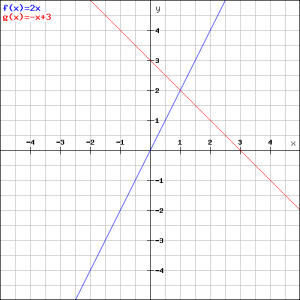# Solution assignment 07 Linear functions and graphs

### Assignment 7

The linegoes through the points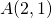and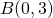and intersects the line: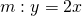in point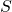.
Calculate the coordinates of.

### Solution

The equation of the lineis not yet known and has to be determined. We can calculate the slope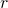ofwith: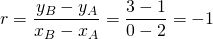Now we have the slope ofwe can calculate the equation and therefore we have to choose one of the points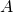and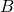. We choose the point, so: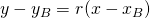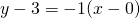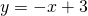We find the-coordinate of the intersection pointby solving: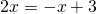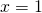and thus we have: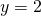The intersection pointhas the coordinates.
Check this in the figure.